# Porous Powders Particle Size Distribution Analysis

Porous powders find application in many industries these days. These range from catalysts to pharmaceuticals; from environmental cleanup to liquid chromatography. Not only is it important to know the pore size distribution of these powders, but it is also crucial to have a reliable particle size distribution analysis of these materials.

In general, these analyses can be performed in much the same way as analyses of nonporous particles. When using laser-scattering particle size analysis, however, a few additional precautions are necessary.

## Laser Light Scattering For Particle Size Analysis

Laser light scattering has been used for particle size analysis for more than 30 years. In 2000, Micromeritics introduced the Saturn DigiSizer High-Definition Digital Laser Particle Size Analyzer, the first such instrument to utilize a CCD detector for high-resolution particle size distribution analysis. Because of the high level of resolution and sensitivity of this analyzer, particle size results are influenced by all types of light scatter phenomenon, including some not related to size but rather to the morphology of the particle.

Not only does light scatter at the surface of the interface between the particle and the suspending medium (often a liquid), it will also scatter as it passes through the pores of a sample. Since the pores are filled with the suspending medium, each time the light passes through a pore, it encounters two additional phase boundaries, and scatters again. The effect directs some light back into the particle, or at very wide angles from the incident light, often away from the light-scattering detector, and definitely in a direction not predicted by spherical particle scattering models regardless of the size of the particles. This phenomenon is similar but not equal to what would be expected for non-transparent particles. In other words, the absence of light at wide scattering angles is similar to the effect of absorption of light within the particle.

But even a higher degree of absorption used in the scattering model cannot account for all of the missing light.

## High Sensitivity Particle Size Analysis

Due to the high level of sensitivity in the particle size analysis capabilities of the Saturn DigiSizer®, the particle size distribution produced for porous materials can be misleading unless that portion of the scattering pattern due to morphology, and not size, is omitted from the particle size calculations. Such can be accomplished by using a feature of the Saturn DigiSizer calculation software. The scattering data can be truncated at a specified scattering angle before the particle size distribution is calculated using nonnegative least squares deconvolution methods. This, combined with allowance for some apparent absorption of light by the particles through the use of an appropriate imaginary refractive index, results in a reliable particle size distribution analysis.

## Imaginary Refractive Index

Simply using a higher imaginary refractive index is not sufficient to account for the missing light, as illustrated in Figure 1. In this case, a porous catalyst (ZSM5) was suspended in water and analyzed four times using the Saturn DigiSizer. A scattering model typical for use with silicas was used to model the expected light scattered by spherical particles to produce a particle size distribution for the powder.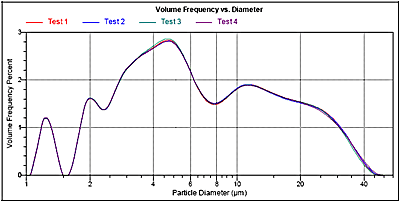Figure 1. Overlay of four analyses of a sample of ZSM5 catalyst powder using the Saturn DigiSizer® 5200. The sample was dispersed in water containing a small amount of sodium metaphosphate. The scattering model used was calculated using a particle real refractive index of 1.45, a particle imaginary refractive index of 0.100i, and a medium real refractive index of 1.331.

Notice that there are a number of modes present at the fine end of the analysis, between 1 and 4 micrometers in diameter. Also note that the fit between this particle size distribution and the measured scattering pattern is not that good at wide angles, as can be seen in the goodness of fit plot for this analysis shown in Figure 2. An imaginary refractive index of 0.100i was used in these calculations, which is much higher than that normally used with transparent materials such as silicas. Since the model intensity is still above the measured intensity in the goodness of fit plot, there is still less light present than predicted by this absorptive model.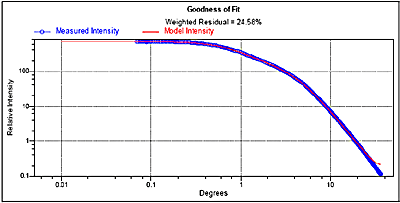Figure 2. Goodness of fit plot between measured scattering pattern and that predicted from the calculated particle size analysis of ZSM5 powder using a scattering model of 1.45, 0.100i in water.

## Truncating the Scattering Pattern

Simply recalculating the results using only a portion of the scattering pattern results in a distribution without most of the additional modes at small diameters, extends to a smaller diameter, and fits the scattering data better. This is because less light is scattered at wide angles than predicted for spherical particles according to the remainder of the scattering pattern. Not using the scattered pattern in the area where light is missing results in a wider and smoother particle size distribution.

Figure 3 shows the result of using scattering data only out to 26.2 degrees. Figure 4 shows the goodness of fit for this calculation, and shows that the weighted residual has improved from 24.58% (Figure 2) to 2.05%.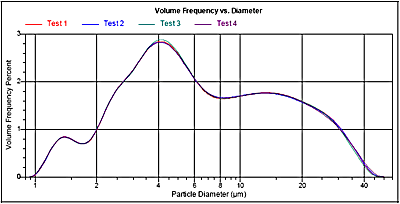Figure 3. Particle size distributions calculated after truncating the scattering pattern at 26.2 degrees.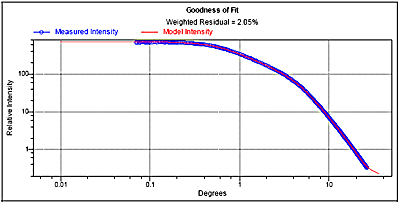Figure 4. Goodness of fit calculated after truncating the scattering pattern at 26.2 degrees.

While this calculation is better, it has not yet been optimized. Further improvement is possible by truncating the scattering pattern at 15.2 degrees, as shown in Figures 5 and 6. Note that the weighted residual has improved to 0.15%.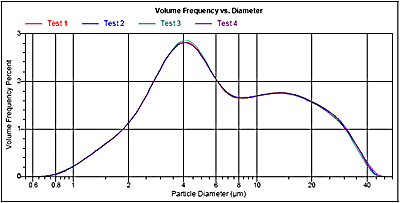Figure 5. Particle size distribution calculated after truncating the scattering pattern at 15.2 degrees.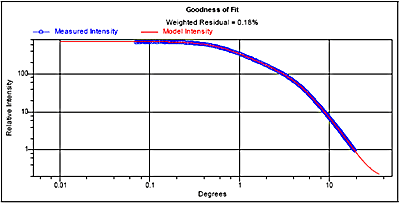Figure 6. Goodness of fit calculated after truncating the scattering pattern at 15.2 degrees.

By truncating the scattering pattern in this manner, the morphology portion of the scattering pattern is not used and only the particle size information remains. Further truncation of the scattering pattern results in loss of information from the fine particles in the distribution. When doing this, the distribution becomes narrower, reversing what had happened up to this point. An overlay of particle size distributions calculated with different ending diameters is shown in Figure 7. Note that the distribution is widest, with the smallest particles detected, when truncating the scattering pattern at 15.2 degrees.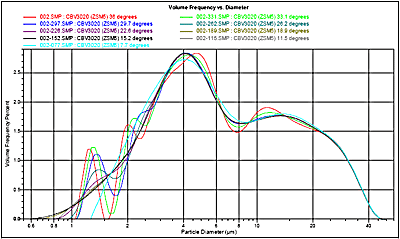Figure 7. Overlay of particle size distribution calculated at different maximum scattering angles.

## Scattering Angles

The angles utilized in this case correspond to the maximum angle at which data are collected for different maximum beam angles. Since the Saturn DigiSizer moves the scattering beam at increments of 5 degrees, those scattering angles corresponding to these incident light positions make natural values for truncating the scattering pattern. Note that the incident angle and the scattering angle are significantly different due to the refractive index of the suspending medium, water in this case. This is because the scattering takes place inside the sample cell (filled with water); however, the detector is outside the cell, so that the light refracts when moving from water to air. Table 1 shows the nominal scattering angle that corresponds to the 10-beam angle positions used by the Saturn DigiSizer for a number of typical dispersing media.

Table 1. Nominal scattering angle equivalent to incident scattering beam angles for the Saturn DigiSizer

 Incident Beam Angle Water RI=1.331 Ethanol RI=1.359 Isopropanol RI=1.376 40 % Sucrose in Water RI=1.400 Odorless Mineral Spirits RI=1.420 Mineral Oil RI=1.467 0 4.0 3.9 3.8 3.8 3.7 3.6 5 7.7 7.6 7.5 7.3 7.2 7.0 10 11.5 11.2 11.1 10.9 10.7 10.4 15 15.2 14.9 14.7 14.4 14.2 13.8 20 18.9 18.5 18.3 18.0 17.7 17.1 25 22.6 22.1 1.8 1.4 21.1 20.4 30 26.2 25.6 25.3 24.8 24.5 23.7 35 29.7 29.0 28.7 28.1 27.7 26.8 40 33.1 32.4 31.9 31.4 30.9 29.9 45 36.0 35.6 35.1 34.5 33.9 32.8This information has been sourced, reviewed and adapted from materials provided by Micromeritics Instrument Corporation.

## Citations

• APA

Micromeritics Instrument Corporation. (2019, December 09). Porous Powders Particle Size Distribution Analysis. AZoM. Retrieved on May 31, 2020 from https://www.azom.com/article.aspx?ArticleID=3206.

• MLA

Micromeritics Instrument Corporation. "Porous Powders Particle Size Distribution Analysis". AZoM. 31 May 2020. <https://www.azom.com/article.aspx?ArticleID=3206>.

• Chicago

Micromeritics Instrument Corporation. "Porous Powders Particle Size Distribution Analysis". AZoM. https://www.azom.com/article.aspx?ArticleID=3206. (accessed May 31, 2020).

• Harvard

Micromeritics Instrument Corporation. 2019. Porous Powders Particle Size Distribution Analysis. AZoM, viewed 31 May 2020, https://www.azom.com/article.aspx?ArticleID=3206.